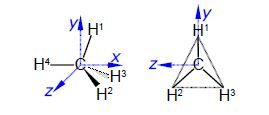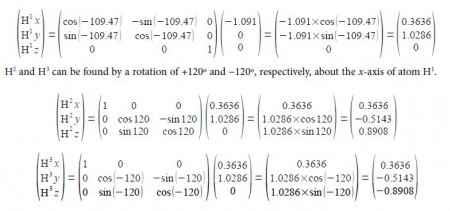Chemistry

# Question Sample: Methane Molecular Structure Analysis

Calculate a set of Cartesian coordinates to 4 significant figures with respect to the origin for the carbon and hydrogen atoms of a methane molecule as shown in below Figure given that the C-H bond distance is 1.091 A and the H-C-H tetrahedral angle is 109.47°, here focus on Methane Molecular Structure.

For ease of calculation note that H1, H2 and H3 form an equilateral triangle and lie in a plane parallel to the yz-axis. Thus find the coordinates for H° which gives H1 by rotation around the z-axis, and then find H2 and H3 by +120° and —120° rotation about the x-axis of H1.Solution

In above Figure the coordinates of C1 are (0, 0, 0) and the coordinates of H4 are (-1.091, 0, 0).

H’ can be found by a clockwise (or negative) rotation of 109.47° about the z-axis of H4.